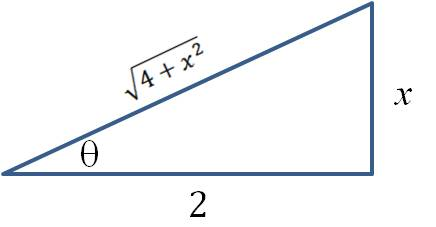## Integrals involving trig substitutions

When is it appropriate to solve an integral with a trig substitution? First of all, keep in mind that a trig substitution doesn’t always work. Even when it does work, you are often left with an integral that will require other techniques such as a u substitution or integration by parts. But if you are willing to put in a little effort (and you know your trig identities), trig substitutions allow you to find the antiderivatives of some rather complicated functions.

There are three conditions that you look for—each a radical term of a particular form in the integrand. Each condition is associated with a different substitution. After you make the substitution, you simplify the integrand and go from there.

 Term Substitution Radical becomes…$\sqrt{a^2-x^2}$$\text{Let } x=a \sin \theta; \, dx=a \cos \theta \,d \theta$$a \cos \theta$$\sqrt{a^2+x^2}$$\text{Let } x=a \tan \theta; \, dx=a \sec^2 \theta \,d \theta$$a \sec \theta$$\sqrt{x^2-a^2}$$\text{Let } x=a \sec \theta; \, dx=a \sec \theta \tan \theta \,d \theta$$a \tan \theta$

Before we look at some example integrals, let’s see why the first radical term above simplifies to$a \cos \theta.$ It’s pretty straightforward if you know your trig identities:$\sqrt{a^2-x^2}=\sqrt{a^2-(a \sin \theta)^2}= \sqrt{a^2-a^2 \sin^2 \theta}= \sqrt{a^2(1- \sin^2 \theta)} =$

……..$\sqrt{a^2 \cos^2 \theta} = a \cos \theta$

Example. Integrate the following:$\text{a.} \displaystyle \int \frac{dx}{\sqrt{1-x^2}} \qquad \text{b.} \int \frac{x^3}{8 \sqrt{4+x^2}}dx \qquad \text{c.} \int x \sqrt{x^2-4} \; dx$

Solutions:

1. (Does this integral look familiar?) Here,$a=1$, so use$x= \sin \theta.$ Using the first line of the table above:
…..$\displaystyle \int \frac{dx}{\sqrt{1-x^2}}= \int \frac{\cos \theta \, d \theta}{\cos \theta}= \int d \theta = \theta +C$
…..
But since$x= \sin \theta, \theta=\sin^{-1} x.$
…..$\therefore \displaystyle \int \frac{dx}{\sqrt{1-x^2}}=\sin^{-1} x+C$
2. Here,$a=2$ and we use line 2 from the table above$(x=2 \tan \theta).$ Note that$x^3=8 \tan^3 \theta.$ Upon substitution,
…..$\displaystyle \int \frac{x^3}{8 \sqrt{4+x^2}}\, dx= \int \frac{8 \tan^3 \theta}{8(2 \sec \theta)}2 \sec^2 \theta \, d \theta = \int \tan^3 \theta \sec \theta \, d \theta$
…..
Hmm. This is going to take a little bit of extra work… Time to pull out some trig identities:
…..$\int \tan^3 \theta \sec \theta \, d \theta = \int \tan^2 \theta \tan \theta \sec \theta \, d \theta = \int (\sec^2 \theta -1) \tan \theta \sec \theta \, d \theta$
…..
Now a u substitution, letting$u= \sec \theta$:
…..$\displaystyle \int (u^2-1) \, du= \frac{1}{3} u^3-u+C= \frac{1}{3} \sec^3 \theta - \sec \theta +C$
…..
How do we get our answer back in terms of$x?$ Draw a triangle that shows how$x$ and$\tan \theta$ are related, then use the Pythagorean theorem to find an expression for$\sec \theta.$ In the triangle below,
…..$x=2 \tan \theta \therefore \sec \theta = \dfrac{\sqrt{4+x^2}}{2}$Substitute into the integral above to get:$\displaystyle \int \frac{x^3}{8 \sqrt{4+x^2}} dx=\frac{1}{3} \sec^3 \theta - \sec \theta +C= \frac{(4+x^2)^{3/2}}{24}- \frac{(4+x^2)^{1/2}}{2}+C$

This can be simplified further by factoring:
…..$\displaystyle \int \frac{x^3}{8 \sqrt{4+x^2}}dx= \frac{(4+x^2)^{3/2}}{24}- \frac{(4+x^2)^{1/2}}{2}+C= \frac{1}{24}(x^2-8) \sqrt{4+x^2}$
…..
Whew!

3. This is a trick question. Even though it fits the condition given in the table (and you could integrate with a trig substitution if you wanted), it’s easier to do this one with a u substitution:$u=x^2-4$ and$du=2x \; dx:$
…..$\displaystyle \int x \sqrt{x^2-4} \, dx= \frac{1}{2} \int \sqrt{u} \, du= \frac{1}{3}u^{3/2}+C= \frac{1}{3}(x^2-4)^{3/2}+C$

The lesson here is to look for u substitutions before you look for trig substitutions.

Calculus
Blue Taste Theme created by Jabox